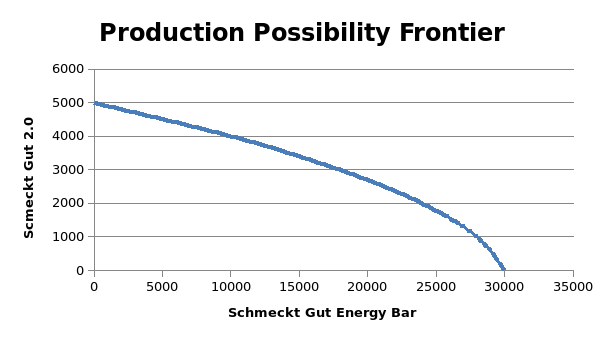# ECON6000 Economic Principles and Decision Making Assignments## PROBLEM A

1) Using the given data, PPF has been drawn with the use of Excel and illustrated below.2) PPF represents the various output possibilities with regards to the given two goods i.e. Schmeckt Gut Energy Bar and Schmeckt Gut 2.0 with regards to using the available resources at maximum efficiency of 100%. Using the given resources available, the production cannot exceed to any point besides the boundary of the PPF curve which has been indicated. As a result, a production of more than 30000 units of Schmeckt Gut Energy Bar is not possible without a change in the factors of production (Dombusch, Fischer & Startz, 2016).

3) In the given case, there has been an increase in the demand of the products owing to which it has exceeded beyond the PPF boundaries (Koutsoyiannis, 2015). This is apparent from the fact that the total demand is 4000 Schmeckt Gut 2.0 along with 20000 units of Schmeckt Gut Energy Bar. However, under the given PPF, it is apparent that with a production level of 4000 Schmeckt Gut 2.0, maximum production level of 10000 units of Schmeckt Gut Energy Bar. Clearly, there would be a shortfall in this case. As a result, suitable measures need to be taken for meeting the increased demand and supply these measures are stated below (Dombusch, Fischer & Startz, 2016).

a) 1) One possibility to meet this increase demand is to improve the technology of production which can be potentially done through the use of automatic machines which would free up labour and also result in higher production levels.

2) Another possibility is to increase the resources devoted to the production of the above two products which could be potentially in the form of capital, land, labour. In case any capital or labour may not be available internally, the same can be brought from outside also in order to meet the increase demand.

3) For meeting the increase demand in the short term, importing from outsider could be an option especially in the short run. This is based on the assumption that surplus is available in the neighbouring region and hence the same can be supplied at a short notice.

### b) The sustainability of the three proposals is discussed as indicated below.

1) The shift in technology is a sustainable means as once done, this shift could be permanent and thus tend to shift the PPF in the outward direction which would indicate enhanced production capabilities and continuous higher level of production.

2) The use of additional resources especially if provided internally would also be sustainable to the extent that the incremental resources are not withdrawn. These would also lead to outward shift in the PPF which would indicate enhanced production capabilities for the combination of the given two products.

3) The importing based measure may not be sustainable since there is dependence on the other country. It is essentially a short term measure aimed to meet the increased demand from District D with the anticipation of working out sustainable measures if the increased consumption is expected to stay (Koutsoyiannis, 2015).

### PROBLEM B

1) In order to estimate the equilibrium price and quantity, it is imperative to equate the demand and supply which is carried out below.

At the equilibrium price, QD=QS = Q, hence

800-2Q = 200 + Q

Solving the above, we get Q = 200

Hence, the equilibrium quantity of the energy bars local market in Industria is 200 units.

Demand equation is P = 800 - 2Q

Putting Q = 200, we get P = \$ 400

Hence, the equilibrium price of the energy bars local market in Industria is \$ 400.

2) It is apparent that from the demand equation that the slope is negative which is in line with the law of demand. As per the law of demand, price and demand tend to be inversely proportional. This implies that if price is increased, there would be a decrease in demand. Similarly, when there is a decrease in the price, then quantity demanded would increase. In the given case, since the price of energy bar is increased by \$ 1, hence the corresponding demand would be lowered in accordance with the demand equation where instead of P, (P+1) would be used (Besanko & Braeutigam, 2014).

Also, it is apparent that the supply equation has a positive slope owing to law of supply. As per this, piece and demand tend to be directly proportional. This implies that if the price is increased, there would be an increase in supply. Similarly, when there is a decrease in the prices, then quantity supplied would also decrease. In the given case, since the price theory of energy bar is increased by \$ 1, hence the corresponding supply would be increased in accordance with the supply equation where instead of P, (P+1) would be used (Pindyck & Rubinfeld, 2014).

#### References

1. Besanko, D. & Braeutigam, R. (2014) Microeconomics,New York: John Wiley & Sons.

Dombusch, R, Fischer, S & Startz, R (2016).Macroeconomics (10th ed) New York: McGraw Hill Publications

1. Koutsoyiannis, A. (2015). Modern Macroeconomics(4thed), London: Palgrave McMillan
2. Pindyck, R. & Rubinfeld, D. (2014) Microeconomics, London: Prentice-Hall Publication